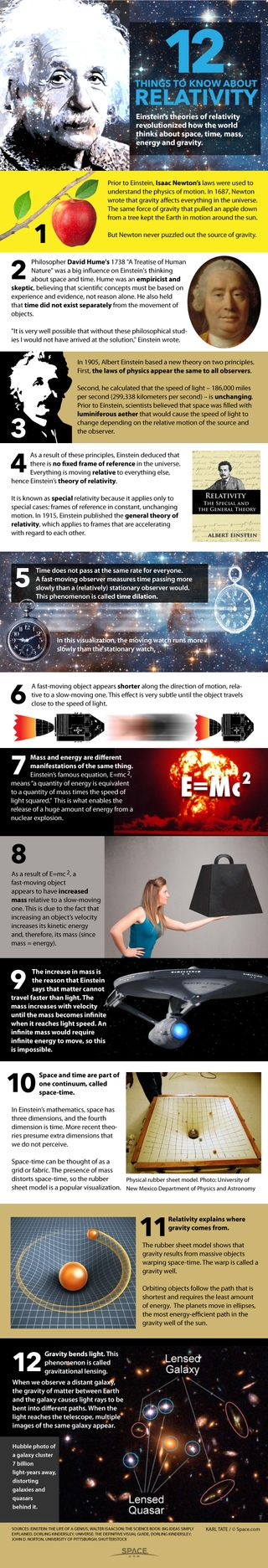# Einstein's Theory of Relativity Explained (Infographic)The theories of relativity by Albert Einstein revolutionized how the world thinks about space, time, mass, energy and gravity.

FULL STORY: General Relativity at 100: Einstein's Famous Theory Has Aged Well

Prior to Einstein, the laws of Isaac Newton were used to understand the physics of motion. In 1687, Newton wrote that gravity affects everything in the universe. The same force of gravity that pulled an apple down from a tree kept the Earth in motion around the sun.

But Newton never puzzled out the source of gravity.

Reference: Einstein's Theory of General Relativity

Philosopher David Hume's 1738 "A Treatise of Human Nature" was a big influence on Einstein's thinking about space and time. Hume was an empiricist and skeptic, believing that scientific concepts must be based on experience and evidence, not reason alone. He also held that time did not exist separately from the movement of objects.

"It is very well possible that without these philosophical studies I would not have arrived at the solution," Einstein wrote.

In 1905, Albert Einstein based a new theory on two principles. First, the laws of physics appear the same to all observers.

Second, he calculated that the speed of light – 186,000 miles per second (299,338 kilometers per second) – is unchanging. Prior to Einstein, scientists believed that space was filled with luminiferous aether that would cause the speed of light to change depending on the relative motion of the source and the observer.

As a result of these principles, Einstein deduced that there is no fixed frame of reference in the universe.

Everything is moving relative to everything else, hence Einstein's theory of relativity

It is known as special relativity because it applies only to special cases: frames of reference in constant, unchanging motion. In 1915, Einstein published the general theory of relativity, which applies to frames that are accelerating with regard to each other.

Time does not pass at the same rate for everyone. A fast-moving observer measures time passing more slowly than a (relatively) stationary observer would. This phenomenon is called time dilation.

A fast-moving object appears shorter along the direction of motion, relative to a slow-moving one. This effect is very subtle until the object travels close to the speed of light.

Mass and energy are different manifestations of the same thing. Einstein's famous equation, E=mc^2, means "a quantity of energy is equivalent to a quantity of mass times the speed of light squared."  This is what enables the release of a huge amount of energy from a nuclear explosion.

As a result of E=mc^2, a fast-moving object appears to have increased mass relative to a slow-moving one. This is due to the fact that increasing an object’s velocity increases its kinetic energy and, therefore, its mass (since mass = energy).

The increase in mass is the reason that Einstein says that matter cannot travel faster than light. The mass increases with velocity until the mass becomes infinite when it reaches light speed. An infinite mass would require infinite energy to move, so this is impossible.

Space and time are part of one continuum, called space-time

In Einstein's mathematics, space has three dimensions, and the fourth dimension is time. More recent theories presume extra dimensions that we do not perceive.

Space-time can be thought of as a grid or fabric. The presence of mass distorts space-time, so the rubber sheet model is a popular visualization.

Relativity explains where gravity comes from. The rubber sheet model shows that gravity results from massive objects warping space-time. The warp is called a gravity well.

Orbiting objects follow the path that is shortest and requires the least amount of energy.  The planets move in ellipses, the most energy-efficient path in the gravity well of the sun.

Gravity bends light. This phenomenon is called gravitational lensing. When we observe a distant galaxy, the gravity of matter between Earth and the galaxy causes light rays to be bent into different paths. When the light reaches the telescope, multiple images of the same galaxy appear.